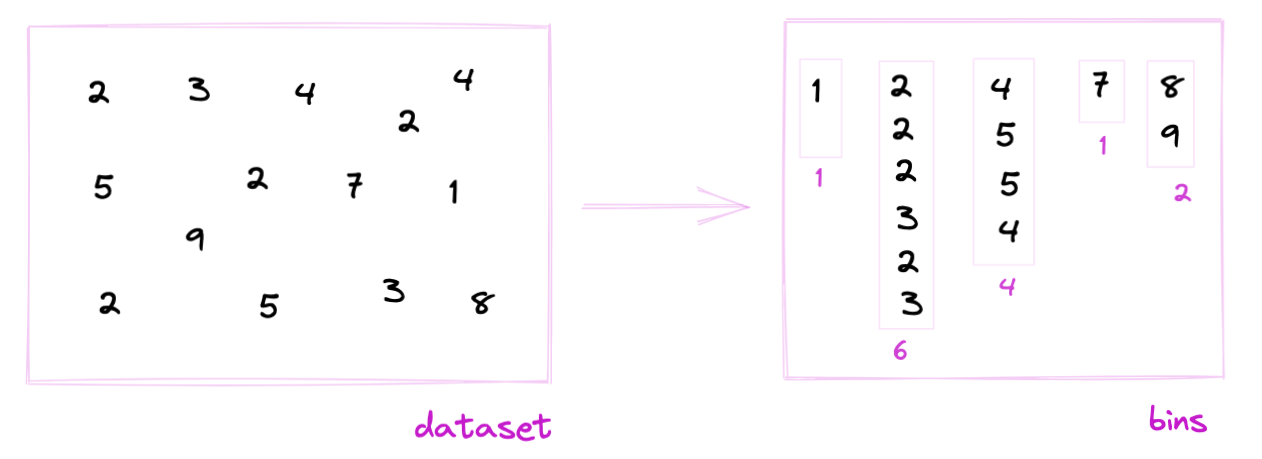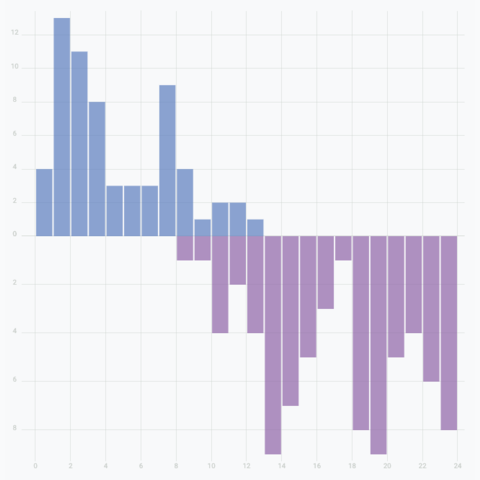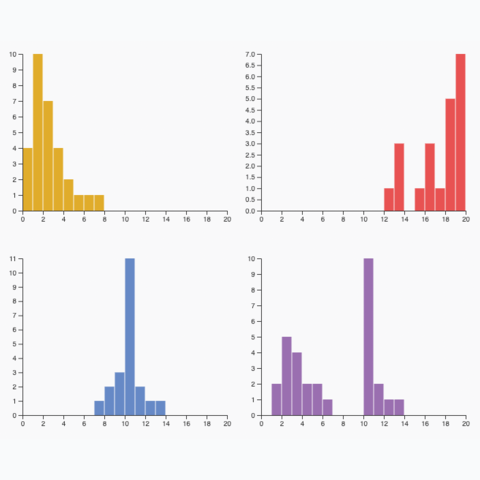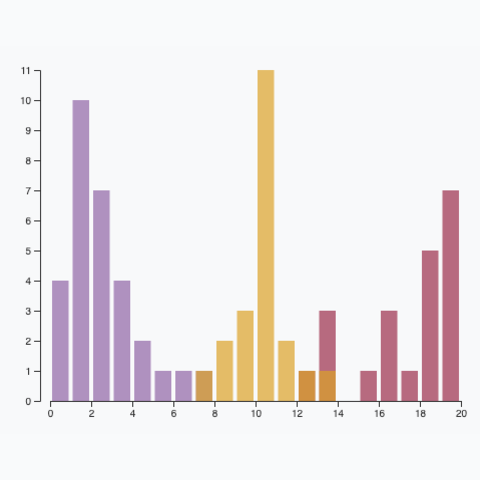# Histogramwith React and d3.jsA histogram is a chart type that shows the distribution of a numeric variable. This page is a step-by-step guide on how to build your own histogram for the web, using React and D3.js.

It starts by describing how the data should be organized and how to initialize the histogram component. It then explains how to compute the buckets composing the histogram. Once this is done, it shows how to render the bars and suggests a few variations. 🙇‍♂️.

## The Data

Building a histogram only requires a set of numeric values.

As a result, the dataset is pretty simple: an `array` of numbers.

Here is a minimal example of the data structure:

``const data = [1, 2, 2, 2, 3, 4, 5, 6, 6, 6, 9]``

## Component skeleton

The goal here is to create a `Histogram` component that will be stored in a `Histogram.tsx` file. This component requires 3 props to render: a `width`, a `height`, and some `data`.

The shape of the `data` is described above. The `width` and `height` will be used to render an `svg` element in the DOM, in which we will insert the histogram.

To put it in a nutshell, that's the skeleton of our `Histogram` component:

``````import * as d3 from "d3"; // we will need d3.js

type HistogramProps = {
width: number;
height: number;
data: number[];
};

export const Histogram = ({ width, height, data }: HistogramProps) => {

// build buckets from the dataset
// build the scales
// build the rectangles

return (
<div>
<svg width={width} height={height}>
// render all the <rect>
</svg>
</div>
);
};``````

It's fundamental to understand that with this code organization, d3.js will be used to prepare the SVG `circle`, but it's React that will render them in the `return()` statement. We won't use d3 methods like `append` that you can find in usual d3.js examples.

## Computing the histogram buckets

### → What is a bin / bucket?

To build a histogram we have to split the data values into a set of buckets. For each bucket, we will count the number of items in it.

This process is called binning. Binning groups discrete samples into a smaller number of consecutive, non-overlapping intervals.Binning is the process of dividing the range of values in a dataset into intervals, and then counting the number of values that fall into each interval.

### → The bin generator

Fortunately, d3.js has a handy `bin()` function for this task. (See the doc.)

The `bin()` function returns a function that is a bin generator. Example:

``````const bucketGenerator = d3
.bin()
.value((d) => d)
.domain([0, 10])
.thresholds([0, 2, 4, 6, 8, 10]);``````

3 arguments are passed to the `bin()` function:

• `value` is the accessor function. For each item of the array, we will pass to the `bucketGenerator`, this is how to get the numeric value of interest.
• `domain` is the lower and upper bounds of the histogram.
• `thresholds` is an array with the limits of each bucket. Note that it can be easily computed from a usual `scaleLinear`.

### → Bucket format

The `bucketGenerator` can be applied to our dummy dataset:

``bucketGenerator(data)``

The result is an array of arrays. Each item represents a bucket. Each bucket is composed of all the values assigned to this bucket. Its `length` is the bucket size, i.e. the future bar height.

Each bin has two additional attributes: `x0` and `x1` being the lower (inclusive) and upper (exclusive) bounds of the bin.

``````[
[x0: 0, x1: 2],
[2, 2, 2, 3, x0: 2, x1: 4],
[4, 5, x0: 4, x1: 6],
[6, 6, 6, x0: 6, x1: 8],
[x0: 8, x1: 10],
[x0: 10, x1: 10],
]``````

Let's transform those buckets in bars 🙇‍♂️!

## Scales

The data wrangling part is done, but we're not ready to draw our bars yet 😢.

Building a histogram requires transforming dimensions (the number of items per bucket and the bucket limits) in positions in pixels. This is done using a fundamental dataviz concept called scale.

D3.js comes with a handful set of predefined scales. `scaleLinear` is what we need for the X and Y axis.

### → X Scale

The X scale is displayed horizontally. It covers the `width` of the `svg` container, and its domain goes from the `min` to the `max` of the dataset.

``````const xScale = d3
.scaleLinear()
.domain([0, 10])
.range([0, width]);

// xScale(0) -> 0 (the left hand side position of the first bin)
// xScale(10) -> width (the right hand side position of the last bin)``````

### → Y Scale

The Y scale is displayed vertically. It shows how many items are available in each bin. To compute it you need to find the bucket with the highest number of items. Something like:

``````const yScale = useMemo(() => {

const max = Math.max(...buckets.map((bucket) => bucket?.length));

return d3.scaleLinear()
.range([height, 0])
.domain([0, max]);

}, [data, height]);``````

## Drawing the bars

Finally! ✨

We can now `map` through the bucket object and draw a rectangle per bucket thanks to the scales computed above.

The code looks like this:

``````const allRects = buckets.map((bucket, i) => {
return (
<rect
key={i}
fill="#69b3a2"
stroke="black"
x={xScale(bucket.x0)}
width={xScale(bucket.x1) - xScale(bucket.x0)}
y={yScale(bucket.length)}
height={height - yScale(bucket.length)}
/>
);
});``````

Remember that the `x` and `y` attributes of the svg `rect` element provide the x and y position of the top left corner of the rectangle (see doc). This is why the rectangle `height` is computed by subtracting `yScale(bucket.length)` from the total `height`.

Values of the dataset as distributed into bins. Bins are represented as rectangles. Data wrangling is made with d3.js, rendering with react.

## Axes

The last step to get a decent chart is to add 2 axes. Otherwise, the bucket bounds are not available, removing all potential insight into the chart.

There are 2 main strategies to add axes to a react chart made with d3.js. This process is extensively described in the axis section.

In the example below, I chose to use the d3 way to render both axes. Note also that a real dataset is used this time, showing the distribution of AirBnB prices on the french riviera.

Adding a X axis with d3 makes the chart much more insightful.

## Responsive Histogram with react

The component above is not responsive. It expects 2 props called `width` and `height` and will render a Histogram of those dimensions.

Making the Histogram responsive requires adding a wrapper component that gets the dimension of the parent `div`, and listening to a potential dimension change. This is possible thanks to a hook called `useDimensions` that will do the job for us.

`useDimensions`: a hook to make your viz responsive
``````export const useDimensions = (targetRef: React.RefObject<HTMLDivElement>) => {

const getDimensions = () => {
return {
width: targetRef.current ? targetRef.current.offsetWidth : 0,
height: targetRef.current ? targetRef.current.offsetHeight : 0
};
};

const [dimensions, setDimensions] = useState(getDimensions);

const handleResize = () => {
setDimensions(getDimensions());
};

useEffect(() => {
return () => window.removeEventListener("resize", handleResize);
}, []);

useLayoutEffect(() => {
handleResize();
}, []);

return dimensions;
}``````

I'm in the process of writing a complete blog post on the topic. Subscribe to the project to know when it's ready.

## Histogram inspiration

If you're looking for inspiration to create your next Histogram, note that dataviz-inspiration.com showcases many examples. Definitely the best place to get ... inspiration!

dataviz-inspiration.com showcases hundreds of stunning dataviz projects. Have a look to get some ideas on how to make your Histogram looks good!

visit

## Variations

Once you've understood how to build a basic histogram with d3 and react, it opens an infinite world of customization. Here are a few examples showing how to add several groups on the same axis or how to use small multiple with histograms to compare distributions.

Click on the overview below to get details and code.Mirror histogramCreate a mirror histogram to compare the distribution of 2 groups in a datasetSmall multipleCreate one panel per group to show its distribution separatelyMultiple groupsA histogram with multiple groups displayed on the same axis.

## Dataset transition

The last step needed for a powerful histogram React component is a proper way to transition between various datasets. When the `data` prop updates, we need a stunning way to transition to the new values.

There are many different strategies to approach this problem. I suggest to rely on the react-spring library that has everything we need to compute spring animations.

Instead of rendering usual `rect` elements, the library provides a `animated.rect` element, that is linked to a `useSpring`hook.

A histogram that smoothly transition from 1 dataset to another

This is how the `Rectangle` component I use looks like:

`Rectangle`: a component that animates the transition of a `rect`
``````import { useSpring, animated } from "@react-spring/web";

type RectangleProps = {
width: number;
height: number;
x: number;
y: number;
};

export const Rectangle = (props: RectangleProps) => {
const { x, y, width, height } = props;

const springProps = useSpring({
to: { x, y, width, height },
config: {
friction: 30,
},
delay: x,
});

if (y === undefined) {
return null;
}

return (
<animated.rect
x={springProps.x}
y={springProps.y}
width={springProps.width}
height={springProps.height}
opacity={0.7}
stroke="#9d174d"
fill="#9d174d"
fillOpacity={0.3}
strokeWidth={1}
rx={1}
/>
);
};``````

Animation in dataviz using React is a big topic. It's impossible to go in depth here! I will publish a dedicated blog post on the topic soon. Please subscribe to the newsletter if you want to be notified.

## Contact

👋 Hey, I'm Yan and I'm currently working on this project!

Feedback is welcome ❤️. You can fill an issue on Github, drop me a message on Twitter, or even send me an email pasting `yan.holtz.data` with `gmail.com`. You can also subscribe to the newsletter to know when I publish more content!